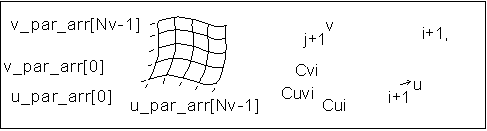Example: Spline Surface Data Format
The parametric spline surface is a nonuniform bicubic spline surface which passes through a grid with tangent vectors given at each point. The grid is curvilinear in uv space.
The tan_cond field, which represents the tangency of the spline surface in the Neutral file format, is an array of two integers. A value of 4 in the tan_cond field indicates that the spline surface is periodic in the given direction. Any other integer value indicates that the spline surface is nonperiodic in the given direction.
A cylindrical spline surface includes a spline surface in its definition.
The following illustration shows a spline surface.Data format:
 u_par_arr[] Point parameters, in the u direction, of size Nu v_par_arr[] Point parameters, in the v direction, of size Nv point_arr[] Array of interpolant points, of size Nu & Nv u_tan_arr[] Array of u tangent vectors at interpolant points, of size Nu & Nv v_tan_arr[] Array of v tangent vectors at interpolant points, of size Nu & Nv uvder_arr[] Array of mixed derivatives at interpolant points, of size Nu & Nv
Parameterization:
Allows for a unique 3 & 3 polynomial around every patch.
There is second-order continuity across patch boundaries.